Function Repository Resource:

# CoefficientMatrix

Returns the coefficient matrix of a system of equations

Contributed by: Dennis M Schneider
 ResourceFunction["CoefficientMatrix"][eqns,vars] returns the coefficient matrix of the system of equations eqns in the variables vars.

## Details and Options

The equations eqns may be a list of equations or a matrix equation.

## Examples

### Basic Examples (3)

Get the coefficient matrix of a linear system:

 In:=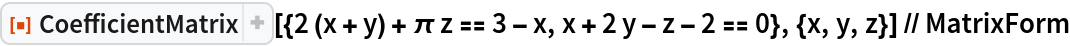Out=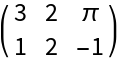Matrix equations are allowed:

 In:=Out=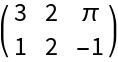In:=Out=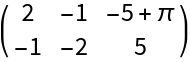### Applications (5)

Find all matrices X that commute with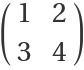. These matrices must satisfy the system of equations:

 In:=Out=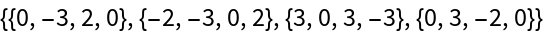We compute the null space of this matrix:

 In:=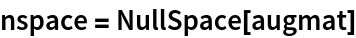Out=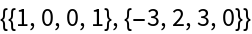A basis for the matrix X:

 In:=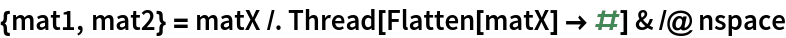Out=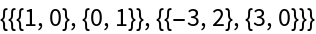Thus, any such matrix must be of the form:

 In:=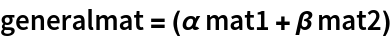Out=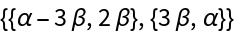Check:

 In:=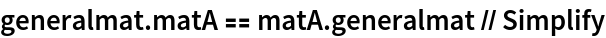Out=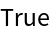## Publisher

Dennis M Schneider

## Version History

• 1.0.0 – 31 July 2019